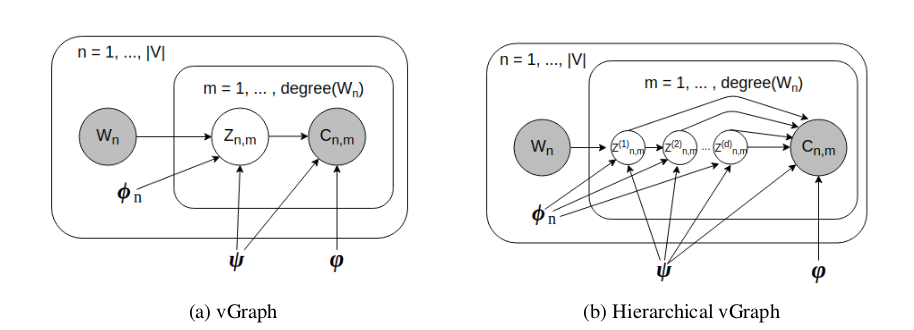# vGraph: A Generative Model for Joint Community Detection and Node Representational Learning

Fan-Yun Sun, Meng Qu, Jordan Hoffmann, Chin-Wei Huang, Jian Tang, NIPS-2019

## Summary

This paper proposes a novel technique for learning node representations and at the same time perform community detection task for the graphical data by creating a generative model using the variational inference concepts.

## Main Contributions

• The authors propose a generative model for jointly learning community membership and node representations, the two tasks even though being highly correlated, studied mostly independently in the previous literature.

• For achieving this, it is assumed that each node can be represented as a mixture of communities, and each community is defined as a multinomial distribution over nodes. Hence we leverage node as well as community embeddings to generate an edge given a node.•  Given a edge between nodes u & v, to obtain a generative model between them, firstly using the fact that each node can be represented a mixture of communities, we find *p(z u)*. Once the community assignment(z) is done and since each community is assumed as a multinomial distribution over nodes, we find the node mostly likely on the other side of the edge determined using the community assignment *p(v z)*.
•  So the generation process can be formulated in a probabilistic way as: **p(c w) = p(c z)p(z w)** (summation over all values of z) where *p(z w)* is the community assignment and can be calculated as a softmax over numerous possible community assignment derived after multiplication of node embeddings with community embeddings. Also *p(c z)* is the node assignment derived by a softmax over the numerous possible nodes derived after multiplication of community and destination node embedding.
•  So as a result we have to maximize the likelihood function **p(c w), however since the process is intractable, and hence we use the concepts of variational inference and rather maximize **ELBO as our loss function.
•  So to do this just like in the case of normal VAEs, we obtain an approximation for the true posterior distribution *p(z c,w)* as *q(z c,w)*, which can further be used to find out the node by using *p(c z). So the ELBO consists of a Cross entropy loss between reconstructed(using w) and original nodes (c*) and KL-divergence loss between the distributions *q(z c,w)* and *p(z w)* (i.e. between the approximated posterior distribution and prior)
•  However to obtain the recontructed node from *q(z c,w)* we need to have a reparametrization trick to make the model end-to-end, however the community assignment are discrete rather than continuous in the case of normal VAEs(the latent variable can be considered as a community assignment in that case). So rather we use Gumbel Reparametrization trick to get a rather discrete assignment while at the same time maintaining the gradient flow of the system.
•  Also to incorporate the tendency of assigning of similar communities to nearby nodes, a regularization is also added incentivizing the distributions *p(z w)* and *p(z c)* to be closer.

## Implementation Details

•  While calculating *p(c z)*, since the softmax is taken over all nodes rendering the technique non-scalable for bigger graphs, negative sampling technique can be used for more efficient calculation in such cases. However usage of negative sampling technique also at the same time leads to slower optimization.
• The usage of Gumbel reparametrization technique leads to an end-to-end model even while leveraging discrete community assignment. More information about the technique can be found here

## Our Two Cents

• The novel technique for performing a joint task by learning a generative model is able to produce SOTA results on various datasets beating out the other technique (ComE) that also performed both the tasks at the same time. Also the usage of negative sampling technique makes the methods extremely scalable to large datasets.

• While the generative model is able to leverage the closeness and neighbours(using the edge information) through its optimization to obtain correct community detection, it is still not able to leverage the node features which can be improved upon in further work.